【LeetCode】从两数之和到四数之和（JS实现）

背景

var twoSum = function(nums, target) {
var arr=[];
for(var i=0;i<nums.length-1;i++){
for(var j=i+1;j<nums.length;j++){
if(nums[i]+nums[j]==target){
arr.push(...[i,j]);
return  arr;
}
}
}
};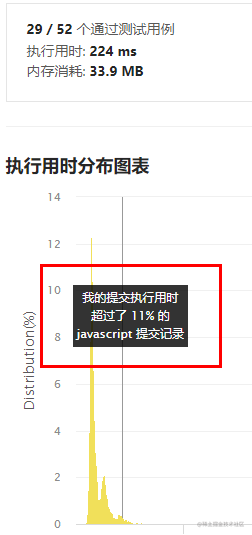（ps：我是菜鸡，思路和想法可能不能让大佬们满意，多多包涵~）

两数之和

例子思路

1. 首先，我们new一个Map，用于存储key和value的映射关系。
2. 之后我们对数组进行遍历。
3. 遍历会有两个情况：
• 这个值之前在Map中出现过，表示，数组中存在重复数据，我们判断这个值的两倍是否是我们要的target。如果是，输出对应的下标，循环结束
• 这个值之前没有在Map中出现过，我们去看 target-当前值 是否存在在Map中，如果存在，则输出对应的下标，循环结束，如果不存在，只需要把当前的 value和key存储在Map中就好。

代码

var twoSum = function(nums, target) {
let map = new Map();
for(let i=0;i<nums.length;i++){
if(map.has(nums[i])){
if( 2*nums[i] === target ){
return [map.get(nums[i]) , i];
}
}else{
if(map.has(target - nums[i])){
return [map.get(target - nums[i]) , i];
}else{
map.set(nums[i] , i);
}
}
}
};结果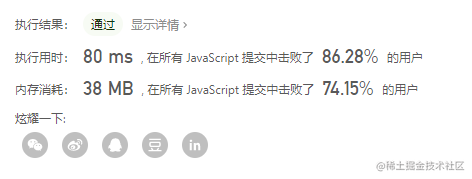三数之和

例子思路

1. 按照上面至理名言所说，我们先排序，得到一个排好序的数组。
2. 之后我们对数组进行遍历
3. 如果nums[i]>0，又因为现在数组是按照从小到大排序，于是，以后不再出现可能的三元组，直接遍历结束
4. 如果nums[i] === nums[i-1],则表示当前数值重复，因不可以出现重复三元组，直接continue跳过本次循环体后续操作
5. 下面我们 设置Left = i+1；设置Right = length-1，就如前面所说的”步步逼近双指针“，我们从L到R进行循环，循环结束条件是Left<R
6. 如果nums[i]+nums[Left]+nums[Right ]>0，则Right左移
7. 如果nums[i]+nums[Left]+nums[Right ]<0，则Left右移
8. 如果nums[i]+nums[Left]+nums[Right ]=0，将[nums[i],nums[Left],nums[Right ]] 追加到结果中去，并且我们这时为了避免重复，需要额外判断:如果nums[Left]==nums[Left+1]，则L++；如果nums[Right ]===nums[Right -1]，则Right --。
9. 返回结果

代码

var threeSum = function(nums) {
let length=nums.length;
nums.sort((a,b)=>a-b);
let res=[];
for(let i=0;i<length;i++){
if(nums[i]>0){
return res;
}
if(i>0&&nums[i]===nums[i-1])
continue;
let Left=i+1;
let Right=length-1;
while(Left<Right){
if(nums[i]+nums[Left]+nums[Right]==0){
res.push([nums[i],nums[Left],nums[Right]]);

while(Left<Right&&nums[Left]==nums[Left+1]){
Left++;
}
while(Left<Right&&nums[Right]===nums[Right-1]){
Right--;
}
Left++;
Right--;
}

else if(nums[i]+nums[Left]+nums[Right]>0){
Right--;
}else{
Left++;
}
}

}
return res
};结果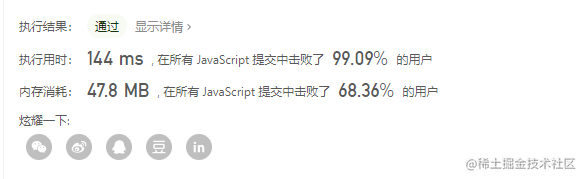四数之和

例子

[
[-1,  0, 0, 1],
[-2, -1, 1, 2],
[-2,  0, 0, 2]
]思路

/**
* @param {number[]} nums
* @param {number} target
* @return {number[][]}
*/
var threeSum = function(nums, target) {
let length=nums.length;
let res=[];
for(let i=0;i<length;i++){
if(nums[i]>target&&nums[i]>0){
return res;
}
if(i>0&&nums[i]===nums[i-1])
continue
let Left=i+1
let Right=length-1
while(Left<Right){
if(nums[i]+nums[Left]+nums[Right]==target){
res.push([nums[i],nums[Left],nums[Right]]);

while(Left<Right&&nums[Left]==nums[Left+1]){
Left++;
}
while(Left<Right&&nums[Right]===nums[Right-1]){
Right--;
}
Left++;
Right--;
}

else if(nums[i]+nums[Left]+nums[Right]>target){
Right--;
}else{
Left++;
}
}
}
return res
};
var fourSum = function(nums, target) {
let length=nums.length;
nums.sort((a,b)=>a-b);
let res=[];
let tempArr = [];
for(let i=0;i<length;i++){
if(nums[i]>target&&nums[i]>0){
return res;
}
if(i>0&&nums[i]===nums[i-1])
continue
tempArr = threeSum(nums.slice(i+1),target-nums[i]);
if(tempArr.length>0){
for(let item of tempArr){
res.push([nums[i],...item]);
}
}
}
return res;
}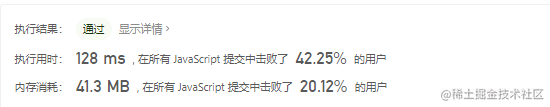1. 首先，我们还是需要对数组进行排序。
2. 因为有四个数，我才用的是 双重循环（first，second）+双指针遍历（Left，Right）的方案，时间复杂度控制在O(n3)。
3. 第一层循环，确定first指向的位置，和三数之和一样，如果nums[first]>target&&nums[first]>0，因为我们直接return.将结果返回。为了不出现重复结果，我们也会判断first>0&&nums[first]===nums[first-1]的结果，如果是true，直接将first右移（countinue）
4. 第二层循环，确定second指向，如果nums[first]+nums[second]>target&&nums[second]>0，我们直接结束循环体（break），因为数组是从小到大排序的，后面的已经不需要再处理。当然，为了不出现重复结果，我们也会判断second>first+1&&nums[second]===nums[second-1]的结果，如果是true，直接second右移（continue）
5. 下面进行双指针遍历，思路和前面的三数之和一样，只不过条件有些许变化。
6. 我们设置 Left = second+1，Right = length-1。双指针遍历结束条件也依然是 Left<Right。
7. 如果nums[first]+nums[second]+nums[Left]+nums[Right]>target，则Right左移
8. 如果nums[first]+nums[second]+nums[Left]+nums[Right]<target，则Left右移
9. 如果nums[first]+nums[second]+nums[Left]+nums[Right]=0，将[nums[first],nums[second],nums[Left],nums[Right]]追加到结果中去，并且我们这时为了避免重复，需要额外判断:如果nums[Left]==nums[Left+1]，则L++；如果nums[Right ]===nums[Right -1]，则Right --。
10. 程序结束

代码

/**
* @param {number[]} nums
* @param {number} target
* @return {number[][]}
*/
var fourSum = function(nums,target){
let length=nums.length;
nums.sort((a,b)=>a-b);
let res = [];
let sumTemp = 0;
for(let first=0;first<length-3;first++){
if(nums[first]>target&&nums[first]>0){
return res;
}
if(first>0&&nums[first]===nums[first-1]){
continue;
}
for(let second=first+1;second<length-2;second++){
if(nums[first]+nums[second]>target&&nums[second]>0){
break;
}
if(second>first+1&&nums[second]===nums[second-1]){
continue;
}
let Left=second+1;
let Right=length-1;
while(Left<Right){
sumTemp = nums[first]+nums[second]+nums[Left]+nums[Right];
if(sumTemp===target){
res.push([nums[first],nums[second],nums[Left],nums[Right]]);
while(Left<Right&&nums[Left]===nums[Left+1]){
Left++;
}
while(Left<Right&&nums[Right]===nums[Right-1]){
Right--;
}
Left++;
Right--;
}
else if(sumTemp>target){
Right--;
}else{
Left++;
}
}
}
}
return res;
}结果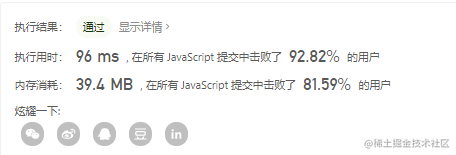（ps：其实一般都是80%左右，90%是运气好）